# The Universal Set

In previous lessons, we learned that a set is a group of objects, and that Venn diagrams can be used to illustrate both set relationships and logical relationships.

Example 1: Given A = {1, 2, 5, 6} and B = {3, 9}, what is the relationship between these sets?

A and B have no elements in common. This relationship is shown in the Venn diagram below.Answer: A and B have no elements in common. These sets do not overlap.

In example 1, A and B have no elements in common. (Each set is shaded with a different color to illustrate this.) Therefore, it is logical to assume that there is no relationship between these sets. However, if we consider these sets as part of a larger set, then there is a relationship between them. For example, consider the single-digit numbers 1 through 9: If {1, 2, 3, 4, 5, 6, 7, 8, 9} is our larger set, then A and B are part of that set. Thus A and B are each a subset of this larger set, called the Universal Set.

Definition: Universal Set is the set of all elements under consideration, denoted by capital. All other sets are subsets of the universal set.

Example 2: Given= {1, 2, 3, 4, 5, 6, 7, 8, 9},  A = {1, 2, 5, 6} and B = {3, 9}, draw a Venn diagram to represent these sets.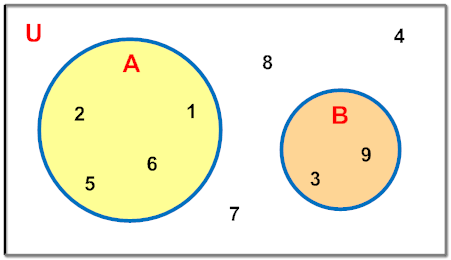Think of a Universal set is the "big picture" It includes everything under consideration, or everything that is relevant to the problem you have. In example 2,and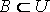Note that subsets A and B do not overlap: These sets are disjoint. The procedure for creating a Venn diagram is as follows;

1. Draw a rectangle and label it U to represent the universal set.
2. Draw circles within the rectangle to represent the subsets of the universe. Label the circles and write the relevant elements in each circle.
3. Write the remaining elements outside the circles but within the rectangle.

Let's look at some more examples.

Example 3: Given= {whole numbers less than 10},  P = {multiples of 3 less than 10} and Q = {even numbers less than 10}, draw a Venn diagram to represent these sets.In example 3, subsets P and Q are overlapping.

Example 4: Given= {whole numbers},  R = {primes numbers less than 12} and S = {even primes}, draw a Venn diagram to represent these sets.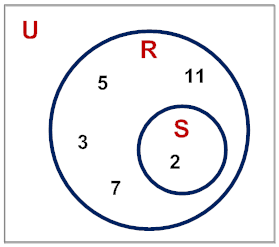In example 4, S is contained within R. This is due to the fact that the number 2 is the only even prime. In addition, the universal set is infinite, since the set of whole numbers goes on forever. Accordingly, we did not include any remaining whole numbers outside the circles and within the rectangle.

Example 5: Given= {animals},  X = {dogs} and Y = {cats}, draw a Venn diagram to represent these sets.In example 5, subsets X and Y do not overlap. Below is a word problem that you may find interesting.

Example 6: In a class of 10 students, some students were selected for the school band, some were selected for the school chorus, some were selected for both, and the rest were selected for neither. Given= {Sam, Kyesha, Derek, Lorrie, Robin, Raúl, Shirley, Nathan, Chris, Dana},  Band = {Sam, Lorrie, Raúl, Derek} and Chorus = {Robin, Derek, Kyesha}, draw a Venn diagram to represent these sets.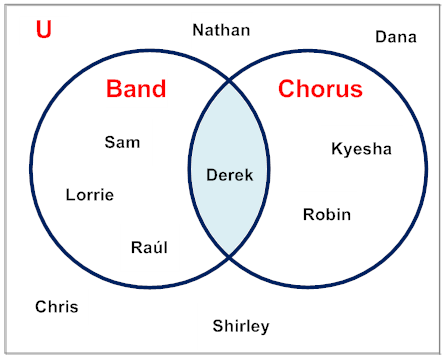In example 6, Band and Chorus are overlapping sets. In addition, Band and Chorus are each a subset of the universal set, which is all the students in the class.

In this lesson, we examined several examples of universal sets with Venn diagrams. In some examples, the sets overlapped and in some they did not. Also included were examples in which one set was contained within the other.

Summary: A universal set is a set containing all elements of a problem under consideration, denoted by capital. A universal set includes everything under consideration, or everything that is relevant to the problem you have. If the universal set contains sets and B, thenand.

### Exercises

 1. If G = {-9, -8, -7, -6} and H = {+8, +2, +7, +4}, then which of the following is the universal set?= {fractions}= {integers}= {irrationals} All of the above. RESULTS BOX:
 2. If X = {Asia, Africa, North America, South America, Antarctica, Europe, Australia}, and Y = {Atlantic, Pacific, Arctic, Indian, Antarctic}, then which of the following could be the universal set?= {oceans}= {countries}= {world} All of the above. RESULTS BOX:
 3. If= {whole numbers}, M = {even numbers} and N = {odd numbers}, then which of the following statements is true?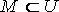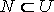M ∩ N = Ø All of the above. RESULTS BOX:
 4. If= {polygons} and A = {quadrilaterals), then which of the following sets does not overlap with A, and is also is a part of? B = {rectangles} C = {triangles} D = {parallelograms} None of the above. RESULTS BOX:
 5. If= {whole numbers less than 40} and P = {1, 4, 9, 16, 25, 36}, then which of the following sets overlaps with P, and is also a part of? Q = {factors of 36} R = {multiples of 4} S = {even primes} None of the above. RESULTS BOX: